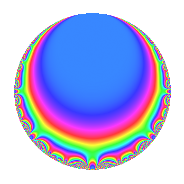# Properties

 Label 28.2.eLevel 28 Weight 2 Character orbit e Rep. character $$\chi_{28}(9,\cdot)$$ Character field $$\Q(\zeta_{3})$$ Dimension 2 Newforms 1 Sturm bound 8 Trace bound 0

# Related objects

## Defining parameters

 Level: $$N$$ = $$28 = 2^{2} \cdot 7$$ Weight: $$k$$ = $$2$$ Character orbit: $$[\chi]$$ = 28.e (of order $$3$$ and degree $$2$$) Character conductor: $$\operatorname{cond}(\chi)$$ = $$7$$ Character field: $$\Q(\zeta_{3})$$ Newforms: $$1$$ Sturm bound: $$8$$ Trace bound: $$0$$

## Dimensions

The following table gives the dimensions of various subspaces of $$M_{2}(28, [\chi])$$.

Total New Old
Modular forms 14 2 12
Cusp forms 2 2 0
Eisenstein series 12 0 12

## Trace form

 $$2q$$ $$\mathstrut -\mathstrut q^{3}$$ $$\mathstrut -\mathstrut 3q^{5}$$ $$\mathstrut -\mathstrut 4q^{7}$$ $$\mathstrut +\mathstrut 2q^{9}$$ $$\mathstrut +\mathstrut O(q^{10})$$ $$2q$$ $$\mathstrut -\mathstrut q^{3}$$ $$\mathstrut -\mathstrut 3q^{5}$$ $$\mathstrut -\mathstrut 4q^{7}$$ $$\mathstrut +\mathstrut 2q^{9}$$ $$\mathstrut +\mathstrut 3q^{11}$$ $$\mathstrut +\mathstrut 4q^{13}$$ $$\mathstrut +\mathstrut 6q^{15}$$ $$\mathstrut -\mathstrut 3q^{17}$$ $$\mathstrut +\mathstrut q^{19}$$ $$\mathstrut -\mathstrut q^{21}$$ $$\mathstrut -\mathstrut 3q^{23}$$ $$\mathstrut -\mathstrut 4q^{25}$$ $$\mathstrut -\mathstrut 10q^{27}$$ $$\mathstrut -\mathstrut 12q^{29}$$ $$\mathstrut +\mathstrut 7q^{31}$$ $$\mathstrut +\mathstrut 3q^{33}$$ $$\mathstrut +\mathstrut 15q^{35}$$ $$\mathstrut +\mathstrut q^{37}$$ $$\mathstrut -\mathstrut 2q^{39}$$ $$\mathstrut +\mathstrut 12q^{41}$$ $$\mathstrut -\mathstrut 8q^{43}$$ $$\mathstrut +\mathstrut 6q^{45}$$ $$\mathstrut +\mathstrut 9q^{47}$$ $$\mathstrut +\mathstrut 2q^{49}$$ $$\mathstrut -\mathstrut 3q^{51}$$ $$\mathstrut -\mathstrut 3q^{53}$$ $$\mathstrut -\mathstrut 18q^{55}$$ $$\mathstrut -\mathstrut 2q^{57}$$ $$\mathstrut -\mathstrut 9q^{59}$$ $$\mathstrut +\mathstrut q^{61}$$ $$\mathstrut -\mathstrut 10q^{63}$$ $$\mathstrut -\mathstrut 6q^{65}$$ $$\mathstrut +\mathstrut 7q^{67}$$ $$\mathstrut +\mathstrut 6q^{69}$$ $$\mathstrut +\mathstrut q^{73}$$ $$\mathstrut -\mathstrut 4q^{75}$$ $$\mathstrut +\mathstrut 3q^{77}$$ $$\mathstrut +\mathstrut 13q^{79}$$ $$\mathstrut -\mathstrut q^{81}$$ $$\mathstrut +\mathstrut 24q^{83}$$ $$\mathstrut +\mathstrut 18q^{85}$$ $$\mathstrut +\mathstrut 6q^{87}$$ $$\mathstrut -\mathstrut 15q^{89}$$ $$\mathstrut -\mathstrut 8q^{91}$$ $$\mathstrut +\mathstrut 7q^{93}$$ $$\mathstrut +\mathstrut 3q^{95}$$ $$\mathstrut -\mathstrut 20q^{97}$$ $$\mathstrut +\mathstrut 12q^{99}$$ $$\mathstrut +\mathstrut O(q^{100})$$

## Decomposition of $$S_{2}^{\mathrm{new}}(28, [\chi])$$ into irreducible Hecke orbits

Label Dim. $$A$$ Field CM Traces $q$-expansion
$$a_2$$ $$a_3$$ $$a_5$$ $$a_7$$
28.2.e.a $$2$$ $$0.224$$ $$\Q(\sqrt{-3})$$ None $$0$$ $$-1$$ $$-3$$ $$-4$$ $$q-\zeta_{6}q^{3}+(-3+3\zeta_{6})q^{5}+(-1-2\zeta_{6})q^{7}+\cdots$$